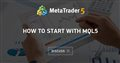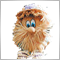Moderator
286078

### Simple Advisor with Stop Loss and Take Profit

An example of a simple Expert Advisor that opens one position with the indicated Stop Loss and Take Profit.

To give a trade order to open a position with Stop Loss and Take Profit, you need to calculate these levels: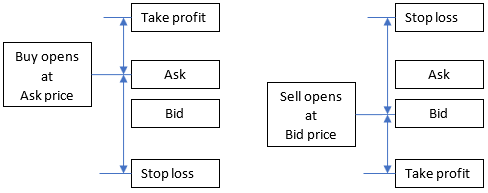Variables declared at the global program level (in the 'header' of the adviser):

```#property version   "1.000"
//---
//---
//--- input parameters```

Input parameters:

I always prefer to set Stop Loss and Take Profit levels in whole types (more precisely in 'ushort')

```//--- input parameters
input ushort   InpStopLoss          = 150;      // Stop Loss, in points (1.00045-1.00055=10 points)
input ushort   InpTakeProfit        = 460;      // Take Profit, in points (1.00045-1.00055=10 points)
input ulong    InpMagic             = 200;      // Magic number
//+------------------------------------------------------------------+
//| Expert initialization function                                   |```

OnTick:

Using PositionsTotal, we determine if there are positions or not. If there are no positions, first we get fresh prices (we do this with SymbolInfoTick). Attention: this is a very simple version - there are not many necessary checks!

```//+------------------------------------------------------------------+
//| Expert tick function                                             |
//+------------------------------------------------------------------+
void OnTick()
{
//---
if(PositionsTotal()==0)
{
MqlTick tick;
if(SymbolInfoTick(Symbol(),tick))
{
}
}
}```

Result: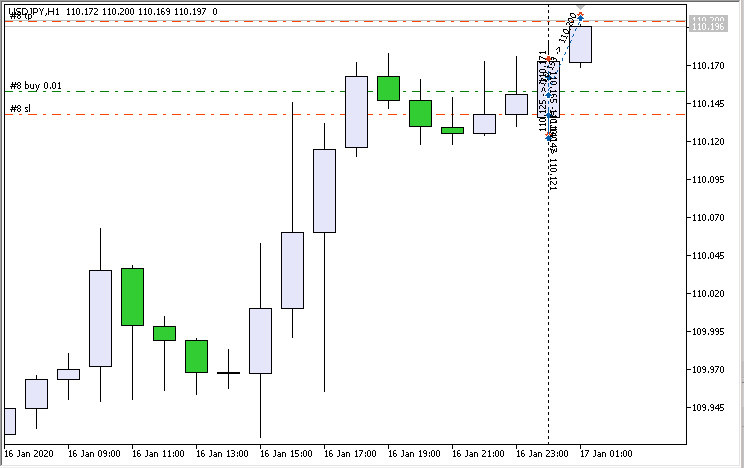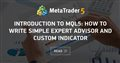Introduction to MQL5: How to write simple Expert Advisor and Custom Indicator
• www.mql5.com
MetaQuotes Programming Language 5 (MQL5), included in MetaTrader 5 Client Terminal, has many new possibilities and higher performance, compared to MQL4. This article will help you to get acquainted with this new programming language. The simple examples of how to write an Expert Advisor and Custom Indicator are presented in this article. We...Moderator
286078

### Creating an iMA indicator handle, getting indicator values

(code: iMA Values on a Chart.mq5)

The main rule: the indicator handle needs to be received once (it is optimal to do this in the OnInit function).

Input parameters and the variable in which the indicator handle is stored:

```//+------------------------------------------------------------------+
//|                                        iMA Values on a Chart.mq5 |
//+------------------------------------------------------------------+
#property version   "1.00"
//--- input parameters
input int                  Inp_MA_ma_period     = 12;          // MA: averaging period
input int                  Inp_MA_ma_shift      = 5;           // MA: horizontal shift
input ENUM_MA_METHOD       Inp_MA_ma_method     = MODE_SMA;    // MA: smoothing type
input ENUM_APPLIED_PRICE   Inp_MA_applied_price = PRICE_CLOSE; // MA: type of price
//---
int    handle_iMA;                           // variable for storing the handle of the iMA indicator
//+------------------------------------------------------------------+
//| Expert initialization function                                   |```

Creating a handle (checking the result)

```//+------------------------------------------------------------------+
//| Expert initialization function                                   |
//+------------------------------------------------------------------+
int OnInit()
{
//--- create handle of the indicator iMA
handle_iMA=iMA(Symbol(),Period(),Inp_MA_ma_period,Inp_MA_ma_shift,
Inp_MA_ma_method,Inp_MA_applied_price);
//--- if the handle is not created
if(handle_iMA==INVALID_HANDLE)
{
//--- tell about the failure and output the error code
PrintFormat("Failed to create handle of the iMA indicator for the symbol %s/%s, error code %d",
Symbol(),
EnumToString(Period()),
GetLastError());
//--- the indicator is stopped early
return(INIT_FAILED);
}
//---
return(INIT_SUCCEEDED);
}```

Getting values (I use the iGetArray generic function). Please note: for the array "array_ma" I use "ArraySetAsSeries" - in this case, in the “array_ma” array, the element with the index “0” corresponds to the rightmost bar on the chart (bar # 0)

```//+------------------------------------------------------------------+
//| Expert tick function                                             |
//+------------------------------------------------------------------+
void OnTick()
{
//---
double array_ma[];
ArraySetAsSeries(array_ma,true);
int start_pos=0,count=3;
if(!iGetArray(handle_iMA,0,start_pos,count,array_ma))
return;

string text="";
for(int i=0; i<count; i++)
text=text+IntegerToString(i)+": "+DoubleToString(array_ma[i],Digits()+1)+"\n";
//---
Comment(text);
}```

The result of work: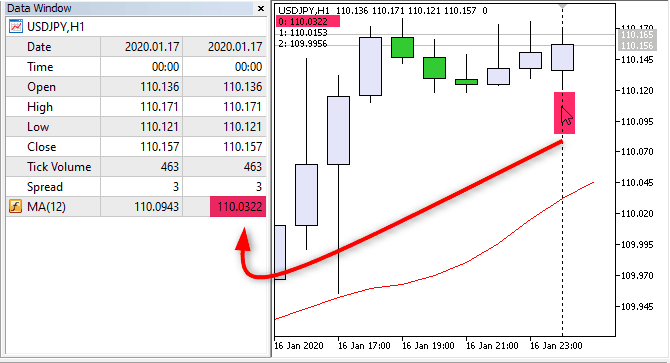Pic. 1. Get value on bar #0, #1, #2

Files:Moderator
286078

Advisor works only on the new bar. The signal to open a position BUY: rsi on bar # 1> 'RSI Level UP'. Signal to open a SELL: rsi position on bar # 1 <'RSI Level DOWN'.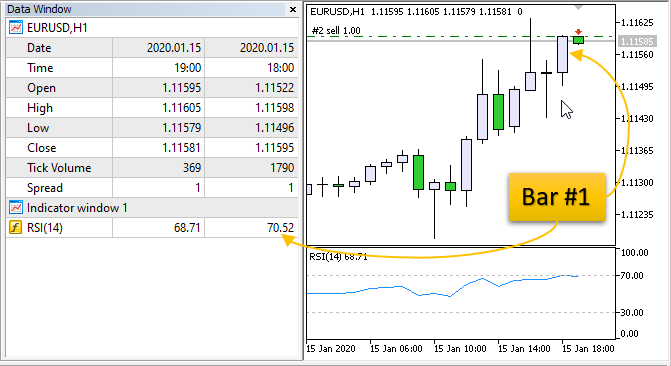```//+------------------------------------------------------------------+
//+------------------------------------------------------------------+
#property version   "1.005"
//---
//--- input parameters
input int                  Inp_RSI_ma_period       = 14;          // RSI: averaging period
input ENUM_APPLIED_PRICE   Inp_RSI_applied_price   = PRICE_CLOSE; // RSI: type of price
input double               Inp_RSI_Level_UP        = 70;          // RSI Level UP
input double               Inp_RSI_Level_DOWN      = 30;          // RSI Level DOWN
//---
int      handle_iRSI;                           // variable for storing the handle of the iRSI indicator
datetime m_prev_bars                = 0;        // "0" -> D'1970.01.01 00:00';
//+------------------------------------------------------------------+
//| Expert initialization function                                   |
//+------------------------------------------------------------------+
int OnInit()
{
//--- create handle of the indicator iRSI
handle_iRSI=iRSI(Symbol(),Period(),Inp_RSI_ma_period,Inp_RSI_applied_price);
//--- if the handle is not created
if(handle_iRSI==INVALID_HANDLE)
{
//--- tell about the failure and output the error code
PrintFormat("Failed to create handle of the iRSI indicator for the symbol %s/%s, error code %d",
Symbol(),
EnumToString(Period()),
GetLastError());
//--- the indicator is stopped early
return(INIT_FAILED);
}
//---
return(INIT_SUCCEEDED);
}
//+------------------------------------------------------------------+
//| Expert deinitialization function                                 |
//+------------------------------------------------------------------+
void OnDeinit(const int reason)
{
//---

}
//+------------------------------------------------------------------+
//| Expert tick function                                             |
//+------------------------------------------------------------------+
void OnTick()
{
//--- we work only at the time of the birth of new bar
datetime time_0=iTime(Symbol(),Period(),0);
if(time_0==m_prev_bars)
return;
m_prev_bars=time_0;
//---
double rsi_1=iRSIGet(1);
if(rsi_1==EMPTY_VALUE)
return;
//---
if(rsi_1>Inp_RSI_Level_UP)
else if(rsi_1<Inp_RSI_Level_DOWN)
}
//+------------------------------------------------------------------+
//+------------------------------------------------------------------+
{
//---
int d=0;
}
//+------------------------------------------------------------------+
//| Get value of buffers for the iRSI                                |
//+------------------------------------------------------------------+
double iRSIGet(const int index)
{
double RSI;
//--- reset error code
ResetLastError();
//--- fill a part of the iRSI array with values from the indicator buffer that has 0 index
if(CopyBuffer(handle_iRSI,0,index,1,RSI)<0)
{
//--- if the copying fails, tell the error code
PrintFormat("Failed to copy data from the iRSI indicator, error code %d",GetLastError());
//--- quit with zero result - it means that the indicator is considered as not calculated
return(EMPTY_VALUE);
}
return(RSI);
}
//+------------------------------------------------------------------+
```

Files:104

Advisor works only on the new bar. The signal to open a position BUY: rsi on bar # 1> 'RSI Level UP'. Signal to open a SELL: rsi position on bar # 1 <'RSI Level DOWN'.

God bless you. If u wanted the trade to be taken on the next bar after signal confirmation had be received. I.E take trade on next bar after signal was confirmed what bit of code would be addedModerator
286078

aodunusi :
God bless you. If u wanted the trade to be taken on the next bar after signal confirmation had be received. I.E take trade on next bar after signal was confirmed what bit of code would be added

I do not understand you.104

I do not understand you.

Once rsi signal has been confirmed, the order is meant to be taken (Buy or Sell). We want to hold that buy order till the next candle forms i.e we don't take trade on the candle we got the trade confirmation at but the next candle that forms / next open price.

Once RSI parameters have been met, hold the buy order and execute it on the next candleModerator
286078

aodunusi :
Once rsi signal has been confirmed, the order is meant to be taken (Buy or Sell). We want to hold that buy order till the next candle forms i.e we don't take trade on the candle we got the trade confirmation at but the next candle that forms / next open price .

Once RSI parameters have been met, hold the buy order and execute it on the next candle

I do not understand: Do you advise me how to trade? Or do you want to delay signal (that is, you want to check the signal not on bar # 1, but on bar # 2)?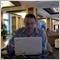Moderator
124443

aodunusi:
Once rsi signal has been confirmed, the order is meant to be taken (Buy or Sell). We want to hold that buy order till the next candle forms i.e we don't take trade on the candle we got the trade confirmation at but the next candle that forms / next open price.

Once RSI parameters have been met, hold the buy order and execute it on the next candle
Means: the bar with signal is closed and new bar opened - and we can open the trade after that (if the previous bar with signal is still existing), right?

It means: trading on close bar.
Yes, the most systems are "trading on close bar" systems.104

Sergey Golubev:
Means: the bar with signal is closed and new bar opened - and we can open the trade after that (if the previous bar with signal is still existing), right?

It means: trading on close bar.
Yes, the most systems are "trading on close bar" systems.
Yes that's what I mean... Once there is a signal wait till the bar closses then trigger buy. Please what code can I use to enable this?Moderator
286078

aodunusi :
Yes that's what I mean... Once there is a signal wait till the bar closses then trigger buy. Please what code can I use to enable this?

You need to redo the code: request data not from one bar, but from two. And check the condition not on one bar - but on two.

It was ( ):

```//+------------------------------------------------------------------+
//| Expert tick function                                             |
//+------------------------------------------------------------------+
void OnTick()
{
//--- we work only at the time of the birth of new bar
datetime time_0=iTime(Symbol(),Period(),0);
if(time_0==m_prev_bars)
return;
m_prev_bars=time_0;
//---
double rsi_1=iRSIGet(1);
if(rsi_1==EMPTY_VALUE)
return;
//---
if(rsi_1>Inp_RSI_Level_UP)
else if(rsi_1<Inp_RSI_Level_DOWN)
}```

Will be:

```//+------------------------------------------------------------------+
//| Expert tick function                                             |
//+------------------------------------------------------------------+
void OnTick()
{
//--- we work only at the time of the birth of new bar
datetime time_0=iTime(Symbol(),Period(),0);
if(time_0==m_prev_bars)
return;
m_prev_bars=time_0;
//---
double rsi_2=iRSIGet(2);
double rsi_1=iRSIGet(1);
if(rsi_2==EMPTY_VALUE || rsi_1==EMPTY_VALUE)
return;
//---
if(rsi_2>Inp_RSI_Level_UP && rsi_1>Inp_RSI_Level_UP)